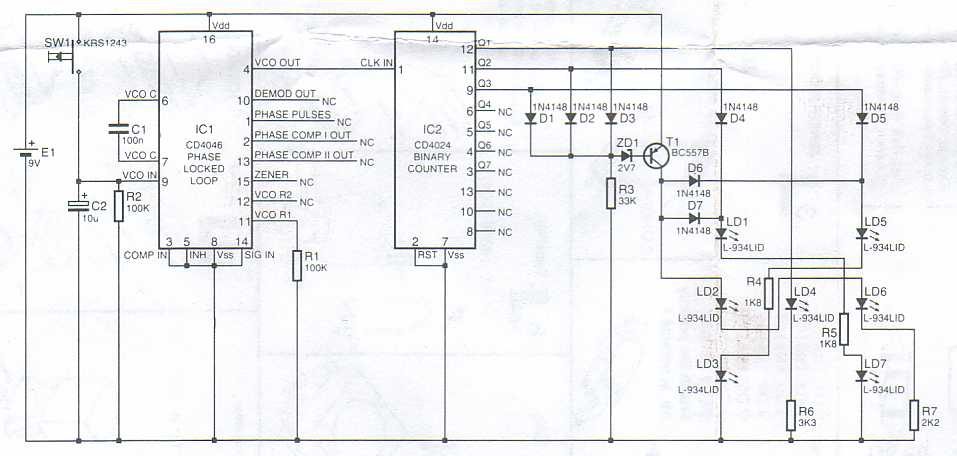# Electronic dice## Partlist:

R1, R2 = 100kOhm
R3 = 33kOhm
R4, R5 = 1,8kOhm
R6 = 3,3kOhm
R7 = 2,2kOhm
D1-D7 = 1N4148
ZD1 = 2,7V
LD1-LD7 = 3mm/Red
C1 = 100nF Ceramic
C2 = 10uF
T1 = BC557
IC1 = 4046
IC2 = 4024